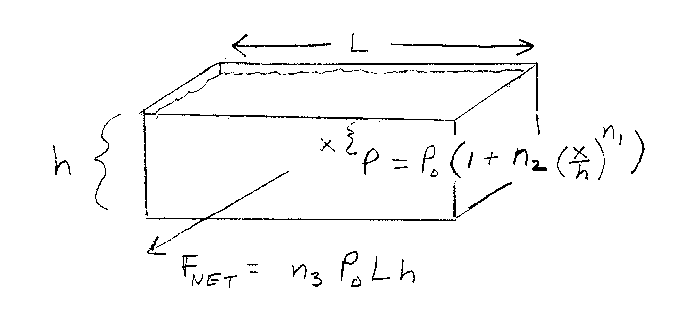Problem A9: Consider the tank containing a strange fluid shown below. The fluid has a depth h. The pressure at the surface of the fluid is P0, and increases with depth x according to the following formula: P = P0(1+n2(x/h)n1). What is the net force on the whole side of the tank? If the net force is F = n3 P0Lh, what is n3? Note that all integers are unitless.n1 = n2 = Input n3:

If you are currently in my class, you can record your grade by entering your name and student ID number (without the leading zeros) below and clicking on "record grade".
 First Name = Last Name = ID = Problem: